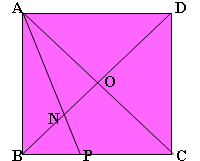#### You may also like### Fitting In

The largest square which fits into a circle is ABCD and EFGH is a square with G and H on the line CD and E and F on the circumference of the circle. Show that AB = 5EF. Similarly the largest equilateral triangle which fits into a circle is LMN and PQR is an equilateral triangle with P and Q on the line LM and R on the circumference of the circle. Show that LM = 3PQ### Triangle Midpoints

You are only given the three midpoints of the sides of a triangle. How can you construct the original triangle?Two ladders are propped up against facing walls. The end of the first ladder is 10 metres above the foot of the first wall. The end of the second ladder is 5 metres above the foot of the second wall. At what height do the ladders cross?

# Look Before You Leap

##### Age 14 to 16Challenge Level

The diagonals of a square meet at $O$.The bisector of angle $OAB$ meets $BO$ and $BC$ at $N$ and $P$ respectively.

The length of $NO$ is $24$.

How long is $PC$?